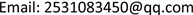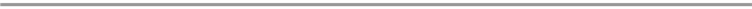﻿ 货物的独立配送路径问题 The Problem of Independent Distribution Path of Goods

Vol. 09  No. 03 ( 2020 ), Article ID: 34568 , 3 pages
10.12677/AAM.2020.93041

The Problem of Independent Distribution Path of Goods

Wei Li

School of Mathematics and Computer Science, Yunnan Minzu University, Kunming YunnanReceived: Feb. 28th, 2020; accepted: Mar. 11th, 2020; published: Mar. 18th, 2020ABSTRACT

By optimizing the distribution of logistics and transportation networks, the cost of distribution can be reduced effectively. The problem of independent path distribution of goods is vehicle routing problem. Vehicle routing problem was first proposed by Dantzig and Ramser in 1959. It is NP-hard problem. In this paper, the Ford-Fulkerson algorithm of maximum flow is used to solve the arc-independent path problems, and then the minimum cost path of minimum cost flow algorithm is used to find R independent paths with minimum total cost. And an optimal solution is obtained to provide new ideas for logistics distribution.

Keywords:Logistics Distribution, Path Planning, Polynomial Algorithm, Independent Path1. 问题描述

2. 算法设计

Step0：取第一个可行流f为零流，取 $c\equiv 1$

Step1：求X增广链。

1.0给s标号 ${l}_{s}=\text{0}$，把所有点规定为未检验点。

1.1若所有已经标号的顶点已经检验完毕，转Step3；否则，任意取一个已经标号却未获得检查的点i，检验与点i关联的弧。 $\forall \left(i,j\right)\in A$，如果 ${x}_{ij}=0$ 并且j没有标号，则给j标号 ${l}_{j}=+i$$\forall \left(j,i\right)\in A$，如果 ${x}_{ji}=1$ 并且j没有标号，那么就给j标号 ${l}_{j}=-i$。当点i的所有关联弧都已经检验完成，则令点i为已经检验，转1.2。

1.2：如果t获得标号，则找到一条增广链，转Step2。否则转1.1。

Step2：对X增广。

2.0：令 $j=t$

2.1：若j的前点标号 ${l}_{j}=0$，即j是s，对X增广结束，消除D中所有顶点的标号，转Step1。否则转2.2。

2.2：如果 ${l}_{i}=+i$，则输出 $\left(i,j\right)$，取 ${x}_{ij}:={x}_{ij}+1$，用点i代替点j，转2.1；如果 ${l}_{i}=-i$，则输出 $\left(j,i\right)$，取 ${x}_{ji}:={x}_{ji}-1$，用点i代替点j，转2.1。

Step3：X的最大流就是有向图D的独立配送路径数，输出配送路径条数K。

Step0：令零流为初始可行流。

Step1：假如 $v\left(x\right)=R$，则X所经过的弧就是有向图D的配送总费用最小的R条独立路径，结束。否则，转Step2。

Step2：把X所经过的弧方向反向，并且令所经弧的权值 $\omega =-\omega$，新的图记为 $H\left(x\right)$

Step3：用Frod算法在有向图 $H\left(x\right)$ 中求最短路P，转Step4。

Step4：使X沿P增广1，获得新的X，转Step1。

Step1：通过算法1求图D中s到t的弧独立路径数K，若 $K\ge R$，转Step2。否则，输出问题无解。

Step2：通过算法2求图D中配送总费用最小的R条独立配送路径。

3. 算法可行性的分析

${{f}^{\prime }}_{ij}=\left\{\begin{array}{l}1,\text{}若\left(i,j\right)\in A\left(P\right)\\ 0,\text{}若\left(i,j\right)\notin A\left(P\right)\end{array}$

$f+{f}^{\prime }$ 是D上流值为 $v+\theta$ 的最小费用流。

The Problem of Independent Distribution Path of Goods[J]. 应用数学进展, 2020, 09(03): 346-348. https://doi.org/10.12677/AAM.2020.93041

1. 1. 梁承姬, 黄涛, 徐德洪, 丁一. 改进遗传算法求解模糊时间窗冷链配送问题[J]. 广西大学学报: 自然科学版, 2016, 41(3): 826-835.

2. 2. Dantzig, G.B. and Ramser, J.H. (1959) The Truck Dispatching Problem. Management Science, 10, 80-91. https://doi.org/10.1287/mnsc.6.1.80

3. 3. 孙智帅. 独立路径问题及其关键顶点和关键弧[D]: [硕士学位论文]. 长沙: 国防科学技术大学, 2012: 17-19.

4. 4. 谢政, 李建平. 网络算法与复杂性理论[M]. 长沙: 国防科技大学出版社, 1995: 177-179.